## Studying linear algebra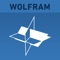# Wolfram Linear Algebra Course Assistant

by Wolfram Group LLC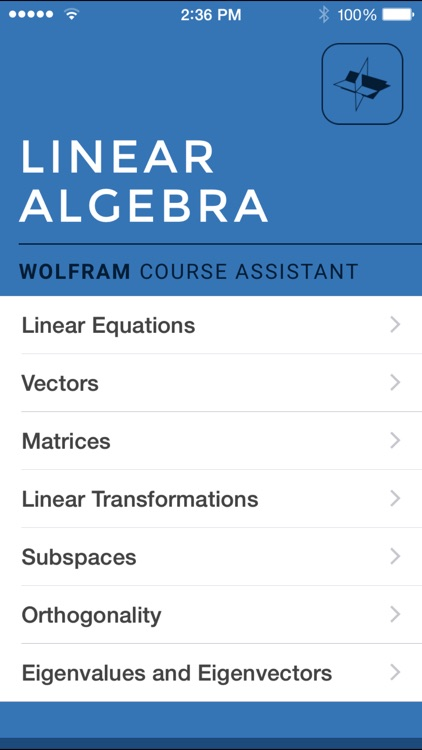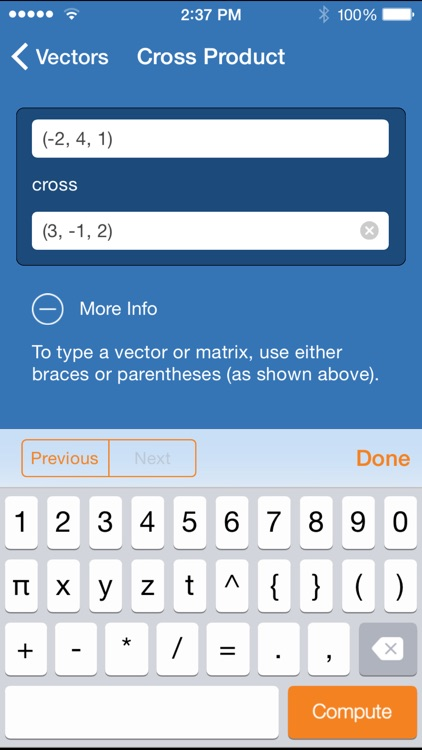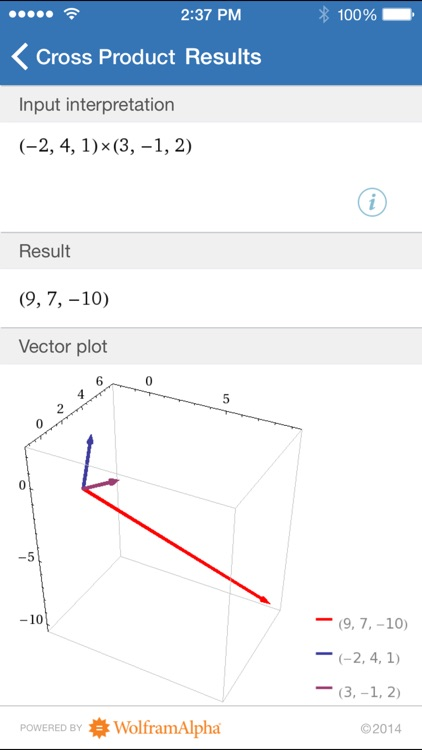Studying linear algebra? Then you need the Wolfram Linear Algebra Course Assistant. This definitive app for linear algebra—from the world leader in math software—will help you work through your homework problems, ace your tests, and learn linear algebra concepts. Forget canned examples! The Wolfram Linear Algebra Course Assistant solves your specific algebra problems on the fly.### App Details

Version
1.3.1
Rating
(1)
Size
12Mb
Genre
Education Reference
Last updated
May 15, 2017
Release date
February 28, 2012

### App Screenshots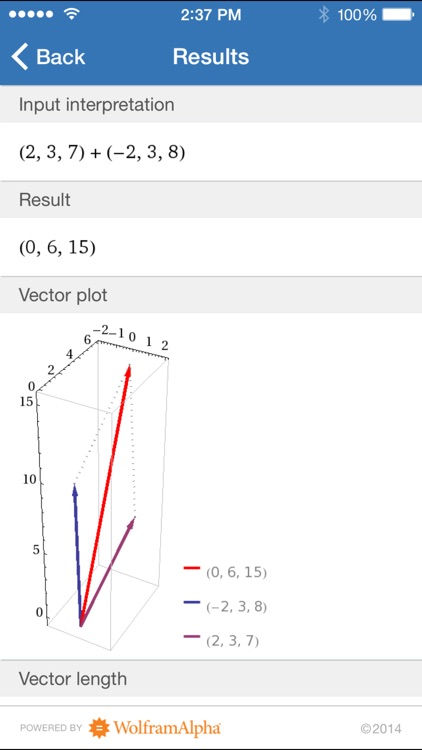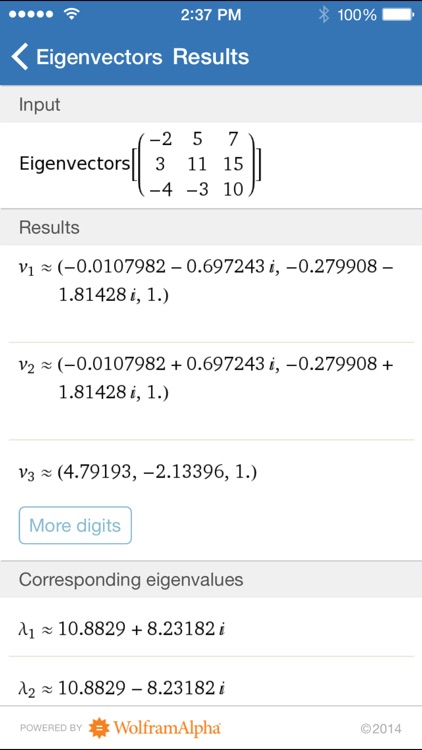### App Store Description

Studying linear algebra? Then you need the Wolfram Linear Algebra Course Assistant. This definitive app for linear algebra—from the world leader in math software—will help you work through your homework problems, ace your tests, and learn linear algebra concepts. Forget canned examples! The Wolfram Linear Algebra Course Assistant solves your specific algebra problems on the fly.

This app covers the following topics applicable to linear algebra:

- Solve an equation or a system of equations
- Add or subtract any two vectors
- Find the cross product or dot product for two vectors
- Find the dimensions, transpose, adjugate, rank, inverse, determinant, and reduced row echelon form of a matrix
- Calculate a matrix product